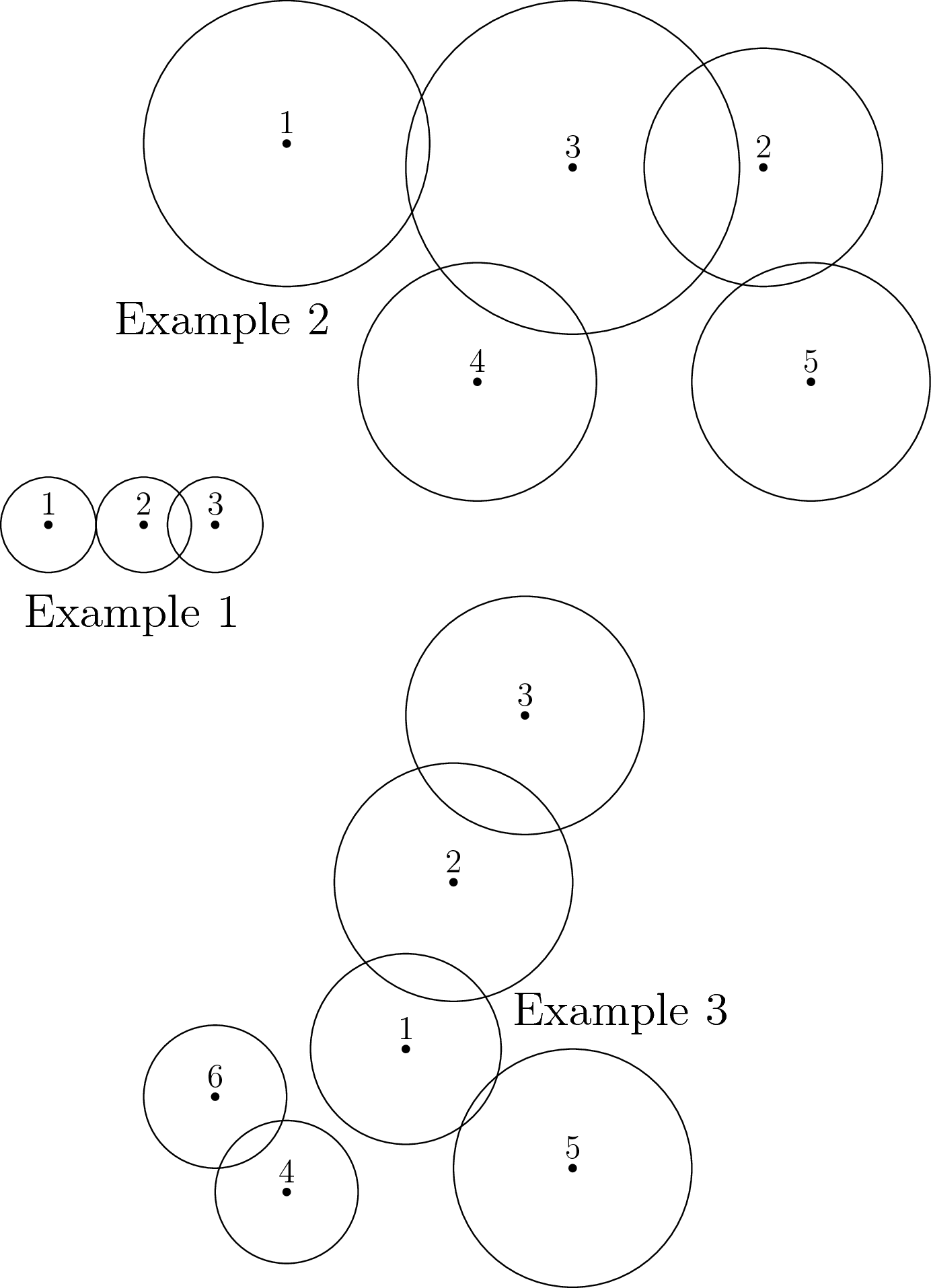# Internet Problem Solving Contest

## Problem T – Turning gears

Jano the mechanic has a lot of gears (cogwheels) of different sizes. One day, he took n of them and assembled a complicated machine. Now he wants to know whether and how fast will gear number n rotate if he attempts to rotate gear number 1.

### Problem specification

There are n gears numbered from 1 to n. All gears are located in the same plane.

Gear i is represented by a circle with center at (xi, yi) and radius ri. All coordinates are integers. The gears are placed so that the center of each circle lies strictly outside all other circles.

Whenever two circles intersect, the corresponding gears are linked to each other correctly. (A single point of contact between two circles does count as an intersection. The size of the intersection does not matter.)

We can describe the rotation of a gear by giving its speed (in full revolutions per second) and its direction of rotation (either clockwise or counterclockwise). Suppose we have two linked gears: one with radius r1 and the other with radius r2. If the first gear rotates at v1 revolutions per second, the other gear will also rotate, but in the opposite direction and possibly at a different speed v2. The speed v2 can be computed as follows: v2 = v1r1/r2.

In a machine with multiple gears the above equation applies to each pair of linked gears. If this would mean a gear has to rotate at two different speeds or directions, it won’t rotate at all.

Jano will attempt to rotate gear number 1 clockwise at 1 revolution per second. How will gear n rotate?

### Input specification

The first line of the input file contains an integer t specifying the number of test cases. Each test case is preceded by a blank line.

Each test case starts with number n (2 ≤ n ≤ 1 000) – the number of gears in the machine. The i-th of the next n lines describes gear i. The description consists of three integers xi, yi and ri – its center and its radius, as described above. You may assume that 0 ≤ xi, yi ≤ 50 000 and 1 ≤ ri ≤ 1 000.

In the subproblem T1 you can make the following additional assumptions about each test case:

• The number of gears will not exceed 200.
• All gears will be directly or indirectly linked to gear 1.
• There will be exactly n − 1 pairs of directly linked gears.

### Output specification

For each test case, output a single line containing the speed and the direction of rotation of gear n. The speed should be expressed as a fraction `p/q` in reduced form. (I.e., the greatest common divisor of p and q should be 1.) The direction should be either “`clockwise`” or “`counterclockwise`”.

If the n-th gear will not rotate at all, print “`does not rotate`” instead.

### ExampleInput:

``````3

3
0 0 2
4 0 2
7 0 2

5
0 10 6
20 9 5
12 9 7
8 0 5
22 0 5

6
8 6 4
10 13 5
13 20 5
3 0 3
15 1 5
0 4 3``````

Output:

``````1/1 clockwise
6/5 counterclockwise
does not rotate``````

In the first test case note that gears 1 and 2 are linked, even though they only touch each other at a single point. Also note that the fraction is printed as “`1/1`”: the denominator is always printed, even if it is 1. The outputs “`2/2`” and “`1`” would both be rejected by the grader.

In the second test case we can compute the answer as follows:

• Gear 1 rotates clockwise at 1 revolution per second (rps).
• Gear 1 is directly linked to gear 3, thus gear 3 rotates counterclockwise at 6/7 rps.
• Gear 3 is also linked to gears 2 and 4. Thus, both of these gears will rotate clockwise. As both of them have the same radius (5), they will both rotate at the same speed: 6/5 rps.
• Gear 5 is linked to gear 2. Therefore, gear 5 will rotate counterclockwise. As gears 2 and 5 have the same radius, gear 5 will also rotate at 6/5 rps.

The third test case does not satisfy the additional constraints for subproblem T1. Hence, this test case can only appear in the subproblem T2. This test case illustrates one of the two main reasons why the answer can be “does not rotate”.